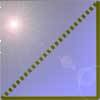#### You may also like### Tri.'s

How many triangles can you make on the 3 by 3 pegboard?### Cutting Corners

Can you make the most extraordinary, the most amazing, the most unusual patterns/designs from these triangles which are made in a special way?### Bracelets

Investigate the different shaped bracelets you could make from 18 different spherical beads. How do they compare if you use 24 beads?

# Egyptian Rope

##### Age 7 to 11 Challenge Level:

Randley School sent in four good solutions.

Naziya said:

I found out that I could make a square with three sections at each side. I also found out that I could make three different triangles: an isoscelos triangle $2$ along the bottom and $5$ up each side, a right angled which had $3$ along the bottom, $4$ up one side and $5$ on the other side and an equilateral with all three sides the same. I could also make two different rectangles, like this: $5, 1, 5, 1$ or $4, 2, 4, 2$.

Matthew and Jordan said:

I made three lots of triangles and I made an isosceles triangle with $2, 5$ and $5$. Then I made an equilateral triangle with three lots of $4$. The last triangle was a right angled triangle with $5, 4$ and $3$. I made two rectangles one had $4, 2, 4, 2$. The last rectangle had $5, 1, 5, 1$. The square had $3, 3, 3, 3$. Then we made a hexagon and it had $2, 2, 2, 2, 2, 2$.

Ffion said:

I made a square with three sections at each side. I made two rectangles one of them was $5$ across and $2$ down, the second one was $4$ across and $2$ down. I made three triangles: a right angled triangle which has $3$ across, $4$ up one side and $5$ on the other side, the isosceles triangle which has $2$ across and $5$ up, the other triangle was the equilateral triangle with all the sides had $4$. I made a hexagon which has $12$ sides.

I'm sure there'll be some rethinking of that last bit - do you know the name of a $12$-sided shape?

Well done everyone.